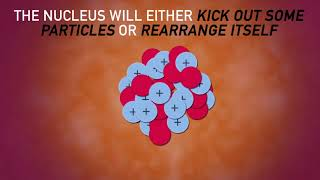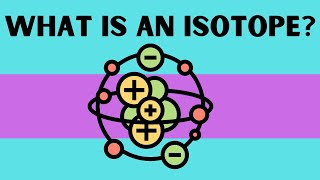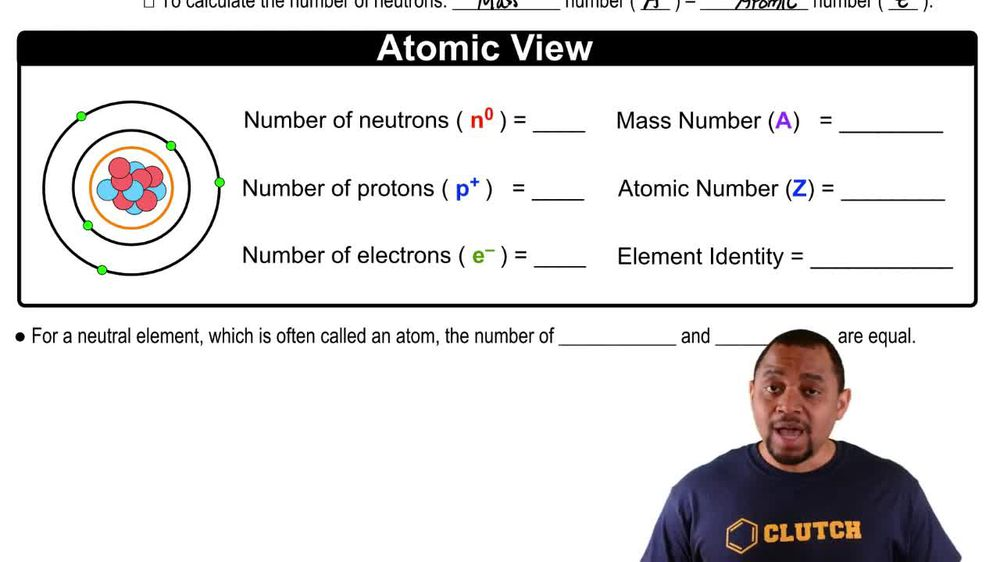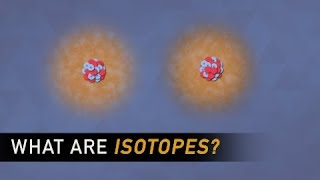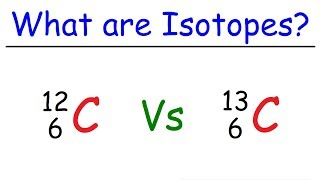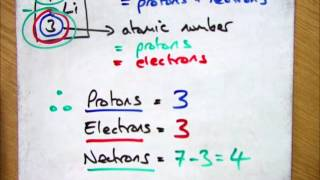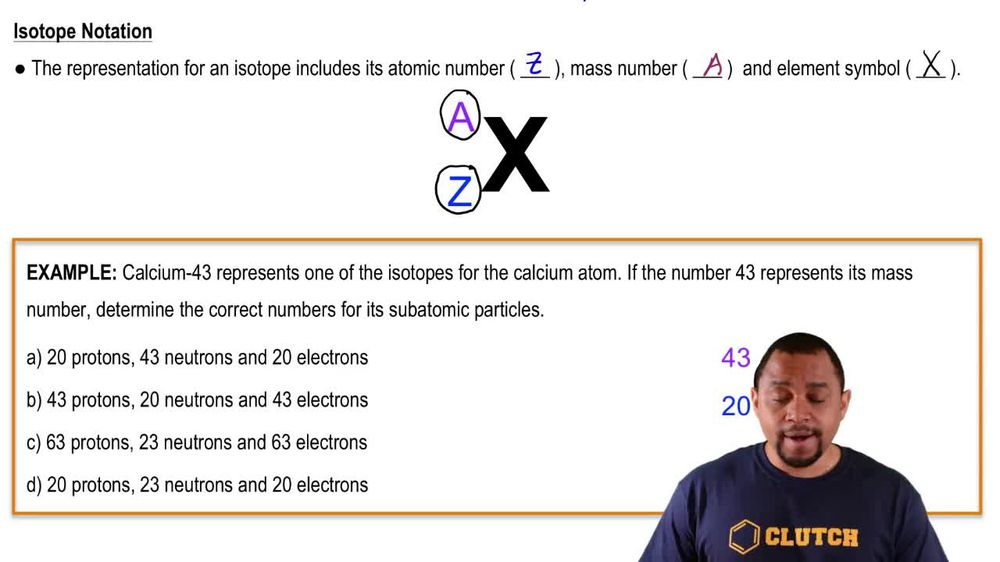Start typing, then use the up and down arrows to select an option from the list.
1. 2. Atoms & Elements2. Isotopes
Problem

# Write isotopic symbols in the form X-A (e.g., C-13) for each isotope. b. the silver isotope with 62 neutrons

Relevant Solution3m
Play a video:
Hello everyone today. We're being asked to identify these symbols, the isotopes below. Using the X. A notation. It's important to recall X. Is representative of the elements symbol and that A represents the mass number for that element. Using this let's get started. A. We have argon and per our periodic table. We can see that are going to have a symbol of our the mass number as noted before is the number of protons I lost the number of neutrons and a species. Since the number of protons, it's equal to its atomic number. We know that argon has an atomic number 18 and therefore has 18 protons. Seeing that we have 21 neutrons, we simply add this to the 18 and we get the mass number 39 a. m. you therefore our notation is a R. 39. Moving on to be we have uranium which is denoted by the atomic element symbol. You put a dash there As we did before, uranium has an atomic number of 92 Meaning it has protons present. The app is two. The number of neutrons to And we get a mass of 323. I am. You. Therefore our symbol notation is you 323. Moving on to see have nitrogen, which is denoted by the symbol in therapeutic table. In nitrogen has a number atomic number of seven Which means it has seven protons present adding to the number of neutrons. We have seven protons plus seven neutrons gives us a total of 14 am you. So therefore augmentation is in dash 14. Last but not least, we have D. Or browning, which hasn't, which is denoted by the symbol B. R. As before. We look at our here on the table and we know that Romeo has an atomic number 35, meaning there's 35 protons present. This is the number of neutrons. Are you fine? And we get e t a m U. Therefore, our notation is BR 80. I hope this helped, and I'll see you in the next one.